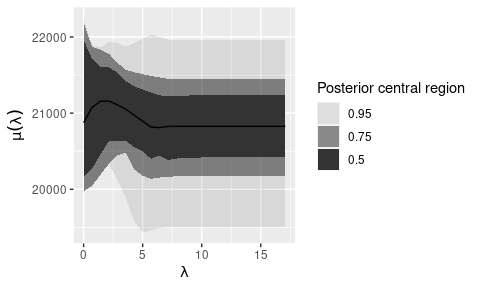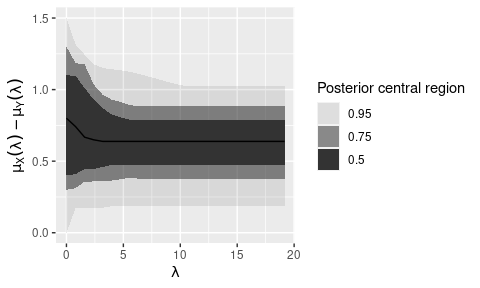# Guide to familial

## Introduction

familal is an R package for testing familial hypotheses. Briefly, familial hypotheses are statements of the form $\mathrm{H}_0:\mu(\lambda)=\mu_0\text{ for some }\lambda\in\Lambda\quad\text{vs.}\quad\mathrm{H}_1:\mu(\lambda)\neq\mu_0\text{ for all }\lambda\in\Lambda,$ where $$\{\mu(\lambda):\lambda\in\Lambda\}$$ is a family of centers. Presently, familial supports tests of the Huber family of centers. The mean and median are members of this family.

To test familial hypotheses, familial uses the Bayesian bootstrap. The Bayesian bootstrap uses weights $$w_1^{(b)},\ldots,w_n^{(b)}$$ ($$b=1,\ldots,B$$) from a uniform Dirichlet distribution in the computation $\mu^{(b)}(\lambda):=\underset{\mu\in\mathbb{R}}{\operatorname{arg~min}}\sum_{i=1}^nw_i^{(b)}\ell_\lambda\left(\frac{x_i-\mu}{\sigma}\right),$ where the Huber loss function $$\ell_\lambda$$ is defined as $\ell_\lambda(z):= \begin{cases} \frac{1}{2}z^2, & \text{if } |z|<\lambda, \\ \lambda|z|-\frac{1}{2}\lambda^2, & \text{otherwise}. \end{cases}$

The above minimization is solved for all values of $$\lambda\in\Lambda=(0,\infty)$$ for $$b=1,\ldots,B$$. The proportion of times that $$\{\mu^{(b)}(\lambda):\lambda\in\Lambda\}_{b=1}^B$$ contains the null value $$\mu_0$$ represents the estimated posterior probability of $$\mathrm{H}_0$$ being true. To map this probability to a decision (accept, reject, or indeterminate), we assign a loss to making an incorrect decision. The decision that minimizes the expected loss under the posterior distribution is the optimal one.

## One-sample tests

Let’s demonstrate the functionality of familial. To perform a test of centers with the Huber family, use the center.test function with argument family='huber' (the default setting). We’ll test whether the velocity of galaxies in the galaxies dataset is different to 21,000 km/sec.

library(familial)
set.seed(1)
x <- MASS::galaxies
test <- center.test(x, mu = 21000)
print(test)
#> -----------------------------------------------
#> familial test of centers with huber family
#> -----------------------------------------------
#> mu = 21000
#> posterior probabilities:
#>    H0    H1
#> 0.542 0.458
#> optimal decision: indeterminate

The output above shows the estimated posterior probabilities for the events $$\mathrm{H}_0$$ and $$\mathrm{H}_1$$. The 54.2% probability assigned to $$\mathrm{H}_0$$ means that the Huber family contained a center equal to 21,000 km/sec in 54.2% of bootstraps. Because neither of the above probabilities is much larger than the other, the test results in an indeterminate outcome. By default, the function will return an indeterminate result when both $$\mathrm{H}_0$$ and $$\mathrm{H}_1$$ have probability less than 0.95. This choice of threshold is analogous to using a frequentist significance level of 0.05.

It’s possible to visualize the posterior using a functional boxplot via the plot function.

plot(test)Rather than specify a null value that is a point, we can specify a null that is an interval. Let’s now test whether the velocity is between 20,500 km/sec and 21,500 km/sec.

center.test(x, mu = c(20500, 21500))
#> -----------------------------------------------
#> familial test of centers with huber family
#> -----------------------------------------------
#> mu = 20500 21500
#> posterior probabilities:
#>    H0    H1
#> 0.959 0.041
#> optimal decision: H0

The test now accepts $$\mathrm{H}_0$$.

## Two-sample tests

familial also supports two-sample testing with paired or independent samples. We’ll now test whether the weight of cabbages in the cabbages dataset is different between two different cultivars. These samples are independent, so we set paired = FALSE.

x <- MASS::cabbages[MASS::cabbages$Cult == 'c39', 'HeadWt'] y <- MASS::cabbages[MASS::cabbages$Cult == 'c52', 'HeadWt']

test <- center.test(x, y, paired = FALSE)
print(test)
#> -----------------------------------------------
#> familial test of centers with huber family
#> -----------------------------------------------
#> mu = 0
#> posterior probabilities:
#>    H0    H1
#> 0.008 0.992
#> optimal decision: H1

The test rejects $$\mathrm{H}_0$$ that the weight of cabbages is the same.

We can also visualize the posterior differences between the family of each cultivar via a functional boxplot.

plot(test)familial also supports one-sided tests. Let’s test whether family treatment (FT) improves the weight of anorexia patients in the anorexia dataset. These samples are paired.

x <- MASS::anorexia[MASS::anorexia$Treat == 'FT', 'Postwt'] y <- MASS::anorexia[MASS::anorexia$Treat == 'FT', 'Prewt']

center.test(x, y, alternative = 'greater', paired = TRUE)
#> -----------------------------------------------
#> familial test of centers with huber family
#> -----------------------------------------------
#> mu = 0
#> posterior probabilities:
#>    H0    H1
#> 0.006 0.994
#> optimal decision: H1

We again reject $$\mathrm{H}_0$$ that FT does not improve the weight of patients.